Discover Free Books That You'll Love!
Receive unbeatable eBook deals in your favorite fiction or non-fiction genres. Our daily emails are packed with new and bestselling authors you will love!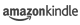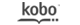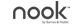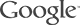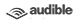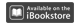# Complexity and Real Computation

## by Lenore Blum, Felipe Cucker, Michael Shub, Steve Smale

Publisher: Springer-Verlag New York, LLC
Pages: 453
Other Format
ISBN: 9780387982816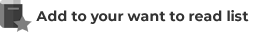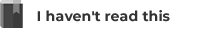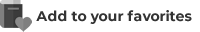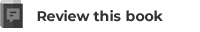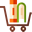## Overview of Complexity and Real Computation

The classical theory of computation has its origins in the work of Goedel, Turing, Church, and Kleene and has been an extraordinarily successful framework for theoretical computer science. The thesis of this book, however, is that it provides an inadequate foundation for modern scientific computation where most of the algorithms are real number algorithms. The goal of this book is to develop a formal theory of computation which integrates major themes of the classical theory and which is more directly applicable to problems in mathematics, numerical analysis, and scientific computing. Along the way, the authors consider such fundamental problems as:
 Is the Mandelbrot set decidable?
 For simple quadratic maps, is the Julia set a halting set?
 What is the real complexity of Newton's method?
 Is there an algorithm for deciding the knapsack problem in a ploynomial number of steps?
 Is the Hilbert Nullstellensatz intractable?
 Is the problem of locating a real zero of a degree four polynomial intractable?
 Is linear programming tractable over the reals? The book is divided into three parts: The first part provides an extensive introduction and then proves the fundamental NP-completeness theorems of Cook-Karp and their extensions to more general number fields as the real and complex numbers. The later parts of the book develop a formal theory of computation which integrates major themes of the classical theory and which is more directly applicable to problems in mathematics, numerical analysis, and scientific computing.

## Synopsis of Complexity and Real Computation

The classical theory of computation has its origins in the work of Goedel, Turing, Church, and Kleene and has been an extraordinarily successful framework for theoretical computer science. The thesis of this book, however, is that it provides an inadequate foundation for modern scientific computation where most of the algorithms are real number algorithms. The goal of this book is to develop a formal theory of computation which integrates major themes of the classical theory and which is more directly applicable to problems in mathematics, numerical analysis, and scientific computing. Along the way, the authors consider such fundamental problems as:
• Is the Mandelbrot set decidable?
• For simple quadratic maps, is the Julia set a halting set?
• What is the real complexity of Newton's method?
• Is there an algorithm for deciding the knapsack problem in a ploynomial number of steps?
• Is the Hilbert Nullstellensatz intractable?
• Is the problem of locating a real zero of a degree four polynomial intractable?
• Is linear programming tractable over the reals? The book is divided into three parts: The first part provides an extensive introduction and then proves the fundamental NP-completeness theorems of Cook-Karp and their extensions to more general number fields as the real and complex numbers. The later parts of the book develop a formal theory of computation which integrates major themes of the classical theory and which is more directly applicable to problems in mathematics, numerical analysis, and scientific computing.

## Reviews of Complexity and Real Computation

There are no reviews yet. Perhaps you can add one!